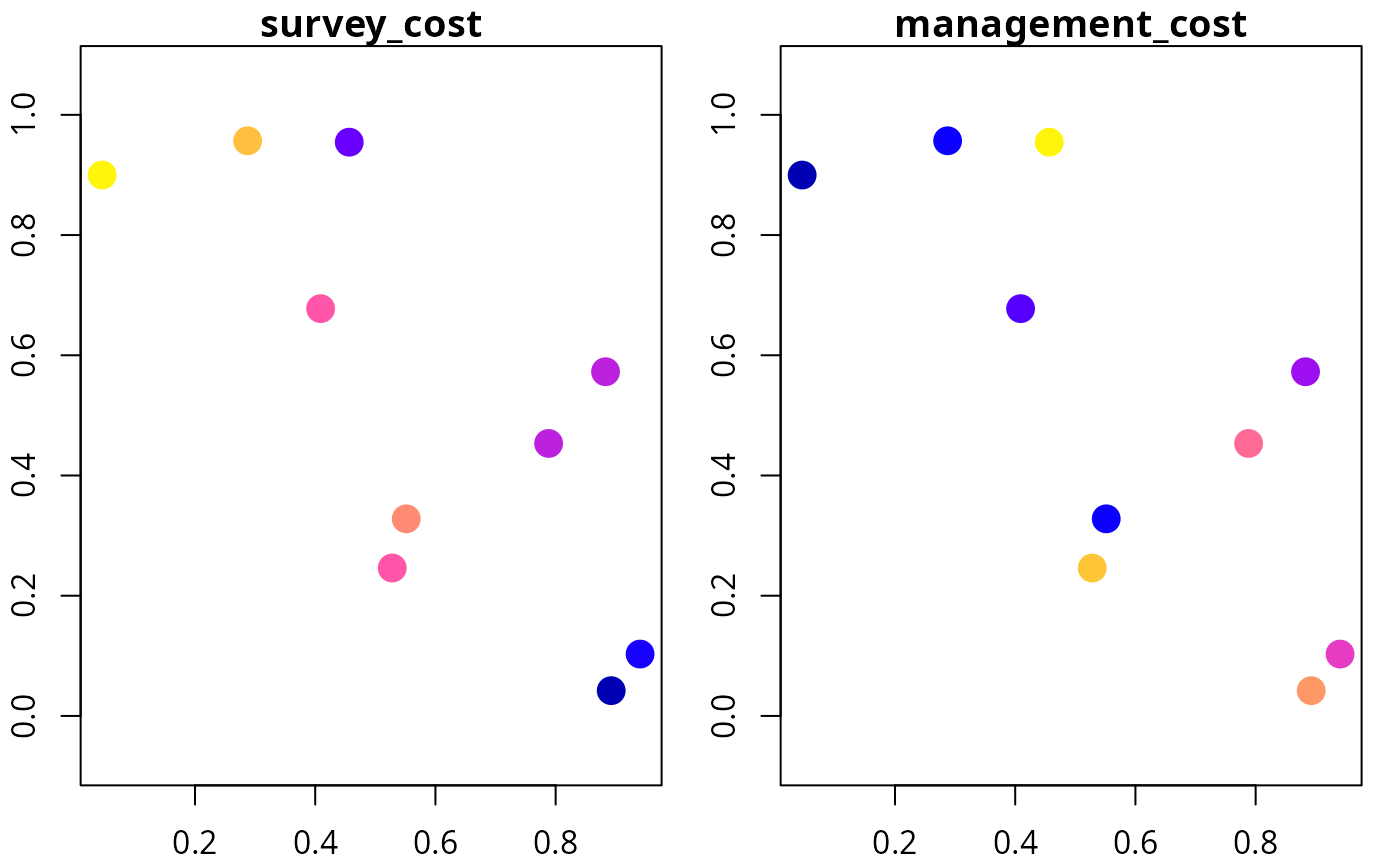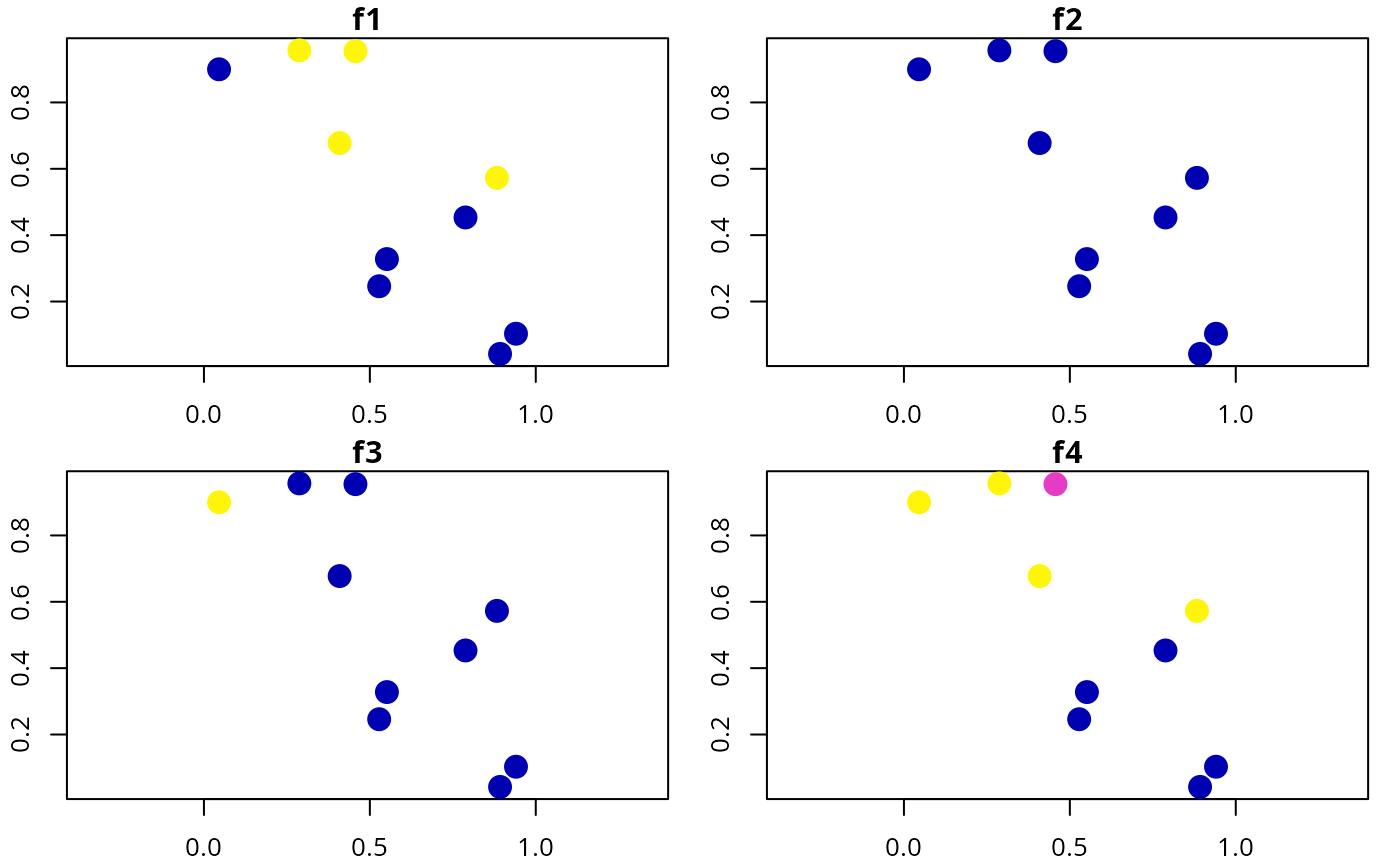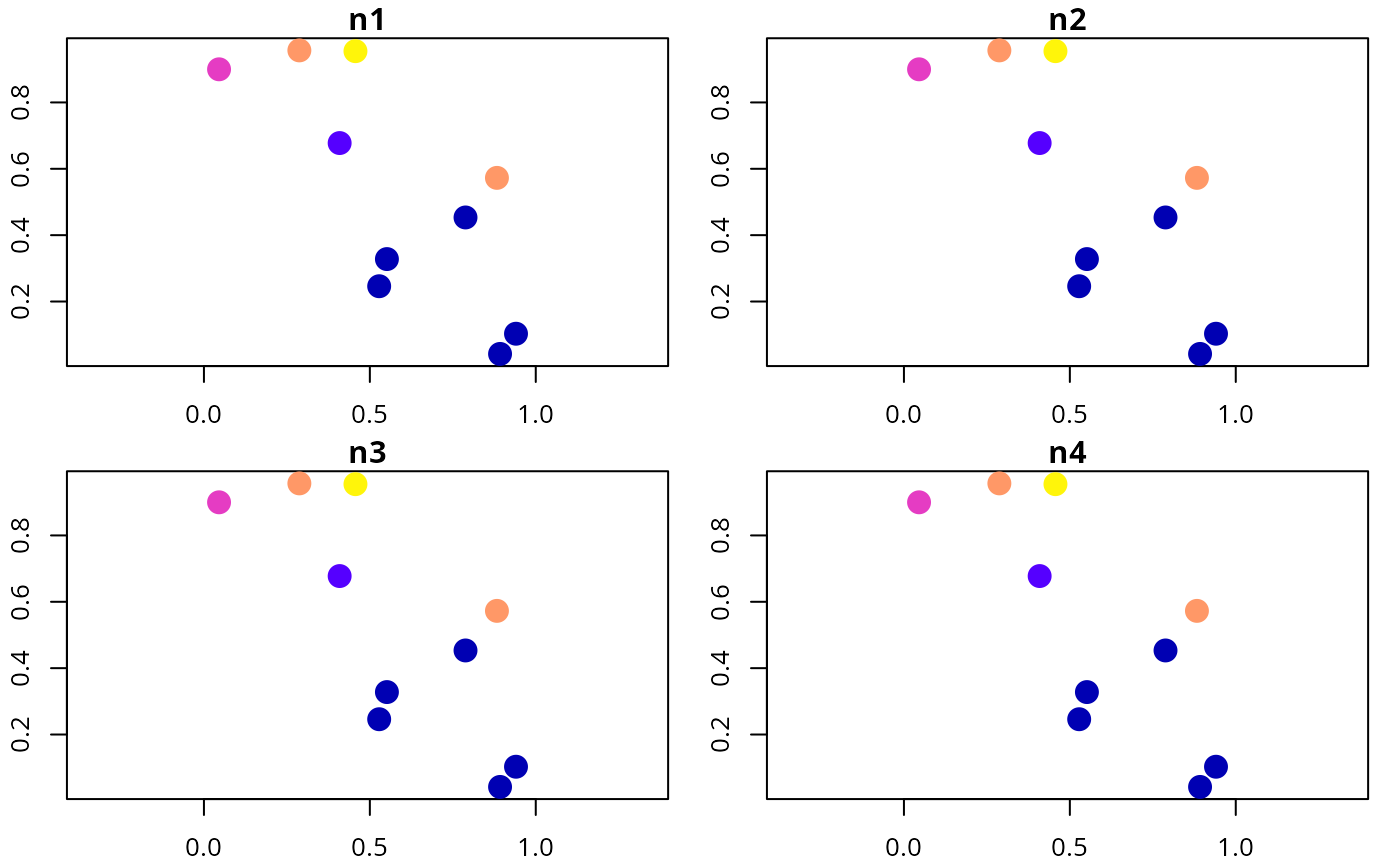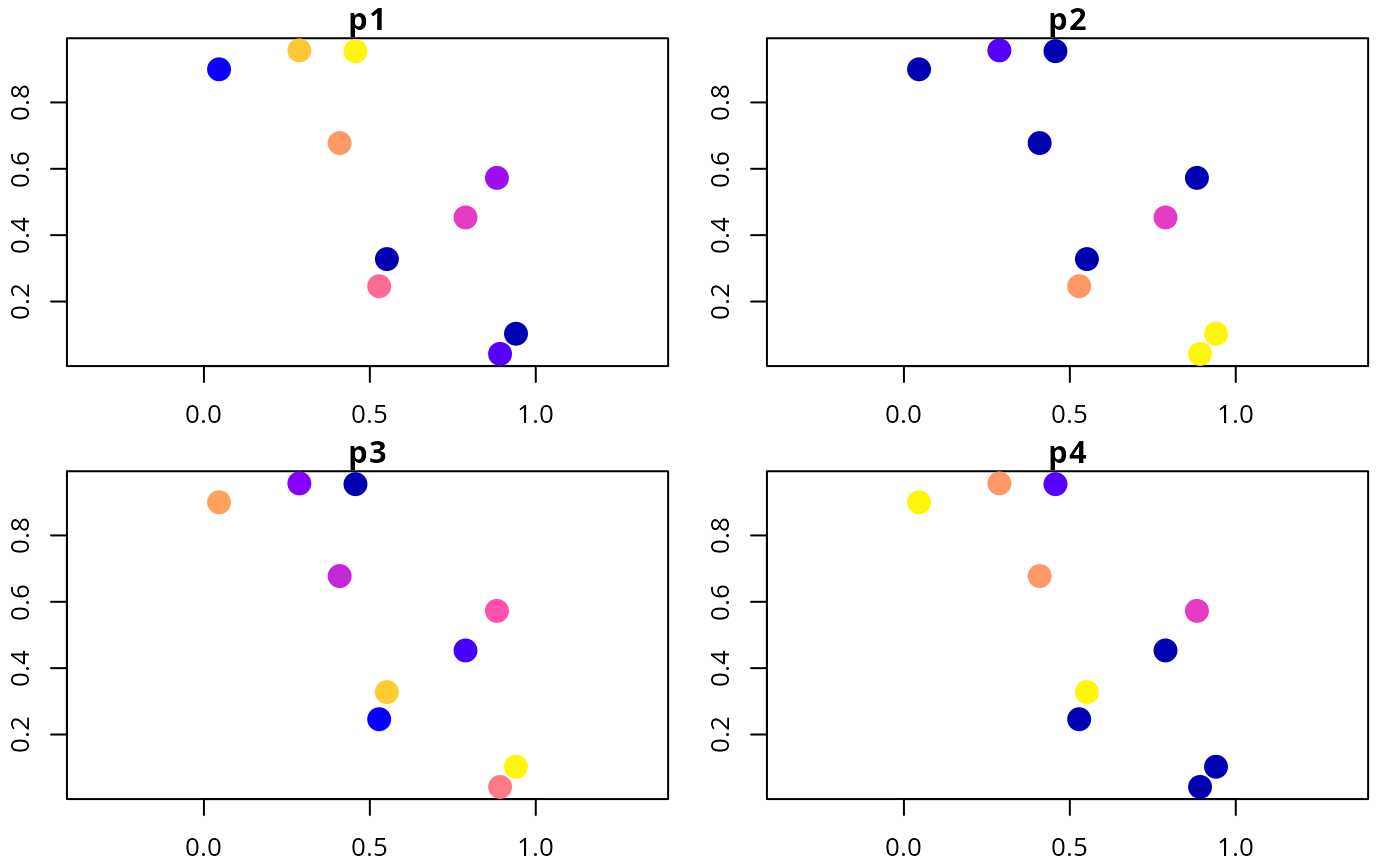Simulate site data for developing simulated survey schemes.

simulate_site_data(
n_sites,
n_features,
proportion_of_sites_missing_data,
n_env_vars = 3,
survey_cost_intensity = 20,
survey_cost_scale = 5,
management_cost_intensity = 100,
management_cost_scale = 30,
max_number_surveys_per_site = 5,
output_probabilities = TRUE
)

## Arguments

n_sites

integer number of sites.

n_features

integer number of features.

proportion_of_sites_missing_data

numeric proportion of sites that do not have existing presence/absence data. Values must be between zero and one.

n_env_vars

integer number of environmental variables for simulating feature distributions. Defaults to 3.

survey_cost_intensity

numeric intensity of the costs of surveying sites. Larger values correspond to larger costs on average. Defaults to 20.

survey_cost_scale

numeric value corresponding to the spatial homogeneity of the survey costs. Defaults to 5.

management_cost_intensity

numeric intensity of the costs of average cost of managing sites for conservation. Defaults to 100.

management_cost_scale

numeric value corresponding to the spatial homogeneity of the survey costs. Defaults to 30.

max_number_surveys_per_site

integer maximum number of surveys per site in the simulated data. Defaults to 5.

output_probabilities

logical value indicating if probability values of occupancy should be output or not. Defaults to TRUE.

## Value

A sf::sf() object with site data. The "management_cost" column contains the site protection costs, and the "survey_cost" column contains the costs for surveying each site. Additionally, columns that start with (i) "f" (e.g. "f1") contain the proportion of times that each feature was detected in each site, (ii) "n" (e.g. "n1") contain the number of of surveys for each feature within each site, (iii) "p" (e.g. "p1") contain prior probability data, and (iv) "e" (e.g. "e1") contain environmental data. Note that columns that contain the same integer value (excepting environmental data columns) correspond to the same feature (e.g. "d1", "n1", "p1" contain data that correspond to the same feature).

simulate_feature_data()

## Examples

# set seed for reproducibility
set.seed(123)

# simulate data
d <- simulate_site_data(n_sites = 10, n_features = 4, prop = 0.5)

# print data
print(d, width = Inf)
#> Simple feature collection with 10 features and 17 fields
#> Geometry type: POINT
#> Dimension:     XY
#> Bounding box:  xmin: 0.0455565 ymin: 0.04205953 xmax: 0.9404673 ymax: 0.9568333
#> CRS:           NA
#> # A tibble: 10 × 18
#>    survey_cost management_cost    f1    f2    f3    f4    n1    n2    n3    n4
#>          <dbl>           <dbl> <dbl> <dbl> <dbl> <dbl> <dbl> <dbl> <dbl> <dbl>
#>  1          26              68     1     0     0   1       3     3     3     3
#>  2          22              93     0     0     0   0       0     0     0     0
#>  3          23              69     1     0     0   1       1     1     1     1
#>  4          22              78     1     0     0   1       3     3     3     3
#>  5          18              81     0     0     0   0       0     0     0     0
#>  6          30              49     0     0     1   1       2     2     2     2
#>  7          23             125     0     0     0   0       0     0     0     0
#>  8          11             103     0     0     0   0       0     0     0     0
#>  9          25              68     0     0     0   0       0     0     0     0
#> 10          21             137     1     0     0   0.4     5     5     5     5
#>        e1      e2      e3    p1    p2    p3    p4              geometry
#>     <dbl>   <dbl>   <dbl> <dbl> <dbl> <dbl> <dbl>               <POINT>
#>  1 -0.145 -0.995  -0.223  0.998 0.004 0.091 0.993 (0.2875775 0.9568333)
#>  2  0.884  0.0440  0.0843 0.949 0.113 0.074 0     (0.7883051 0.4533342)
#>  3  0.794 -0.627  -0.637  0.957 0     0.134 0.993 (0.4089769 0.6775706)
#>  4  0.297 -0.540  -0.402  0.929 0     0.24  0.992 (0.8830174 0.5726334)
#>  5 -1.45   1.65    1.21   0     1     0.994 0     (0.9404673 0.1029247)
#>  6 -1.45  -1.13   -1.22   0.024 0     0.99  1      (0.0455565 0.899825)
#>  7  0.878  0.222   0.312  0.956 0.894 0.059 0     (0.5281055 0.2460877)
#>  8 -1.23   1.42    2.08   0.35  1     0.555 0     (0.892419 0.04205953)
#>  9  0.731  0.761  -0.990  0     0     0.991 1      (0.551435 0.3279207)
#> 10  0.685 -0.814  -0.211  0.999 0     0.02  0.117 (0.4566147 0.9545036)

# plot cost data
plot(d[, c("survey_cost", "management_cost")], axes = TRUE, pch = 16,
cex = 2)# plot environmental data
plot(d[, c("e1", "e2", "e3")], axes = TRUE, pch = 16, cex = 2)# plot feature detection data
plot(d[, c("f1", "f2", "f3", "f4")], axes = TRUE, pch = 16, cex = 2)# plot feature survey effort
plot(d[, c("n1", "n2", "n3", "n4")], axes = TRUE, pch = 16, cex = 2)# plot feature prior probability data
plot(d[, c("p1", "p2", "p3", "p4")], axes = TRUE, pch = 16, cex = 2)